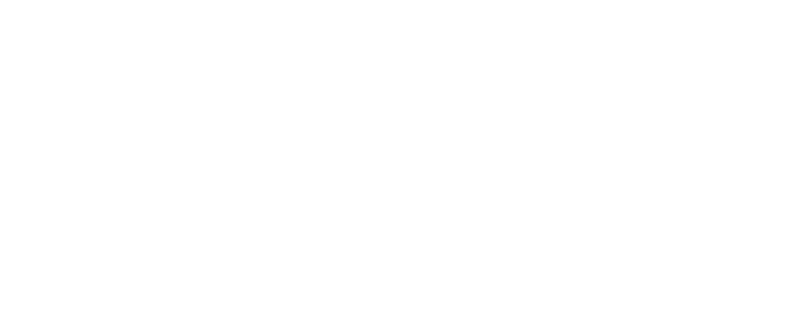### Set - 1

#### Group A

1.

1. What is the x - coordinate of the vertex of the parabola whose equation is $Y = x^{2}+ bx + c, ≠0 ?$
2. If a polynomial f(x) is divided by (x - a), what will be its remainder?

2.

1. Define the discontinuity of a curve of a function.
2. Under what condition, the inverse of a matrix is possible?

3.

1. If the intersection plane is parallel to the axis of the cone then what conic does it form?
2. What is the single equation to represent the pair of lines x + a = 0 and x - a = 0?

4.

1. If $\sin\left( \theta \right) = \sqrt{ \dfrac{ 3 }{ 2 } \phantom{\tiny{!}}}$, what is the value of θ for (0≤ θ ≤ 90)?
2. In which transformation does the point (4, 5) map to (-5, 4)?

## Group B

5.

1. If (x - m) is a factor of $x^{3} - mx^{2}- 4x + m + 12,$ calculate the value of m.
2. Find the common ratio of a geometric sequence whose first term is 2 and the third term is 242.
3. The show has given 4x + 5y ≥ - 20 inequalities on a graph.

6.

1. If p =$\begin{bmatrix}3 & 0 \\0 & 2 \end{bmatrix}$, find $p^{-1}$
2. If matrix P = $\begin{bmatrix}3 & -2 \\4 & 1 \end{bmatrix}$and Q =$\begin{bmatrix}-2 & 1 \\3 & -2 \end{bmatrix}$, find the determinant of the matrix PQ.

8.

1. Show that the lines $y+\sqrt{3x}-4=0$ and $x-\sqrt{3y}=5$ are perpendicular to each other.
2. Find the angle between the pair of straight lines represented by the equation $3x^{2}+7xy+yx^{2}=0$.

9.

1. Prove that: $\frac{1-2sinA.cosA}{2}=sin^{2}(\frac{\pi^{c}}{4}-A)$ $cos20^{o} + cos140^{o}+ cos100^{o} = 0$
2. Solve: 3secθ + 4cosθ   $3secθ + 4cosθ (0^{o}≤ θ ≤ 90^{o})$

10.

1. In the given ΔABC, AB = BC = CA = 6cm. Find the value of $\overline{AB}.\overline{BC}$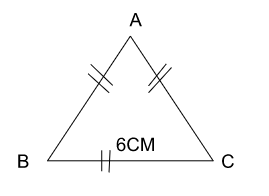1. If $\overline{a}= \frac{4}{3}$, find the vector perpendicular to ā whose length along the x-axis is 6 units.
2. In grouped data, the value of the first quartile is 2m and the quartile deviation is 1m, find the third quartile and coefficient of quartile deviation.

## Group c

11. Sove: $x^{3} - 9x^{2} + 24x - 20 = 0$

12. Find the maximum value of the objective function p(x, y) = 3x + 2y under the following constraints. 2y ≥x - 1, x + y ≤ 4, x ≥ 0 and y ≥ 0.

13. A function f(x) is defined as follows: f(x) = ${kx^{2} when x≤ 2 = 3 when x > 2}$

14. Solve by Cramer's rule:  $\frac{2}{x}+\frac{5}{y}= 1:\frac{3}{x}+\frac{2}{y}=\frac{19}{20}$

15. Find the equation of straight lines passing through the point ( - 1, 4) and making an angle of $45^{o}$with the line 3x + 4y = 12

16. Prove that: $\frac{2sinx}{\cos3x}+\frac{2\sin3x}{cos9x}+\frac{2\sin9x}{\cos27x}= \tan27x-tanx$

17. If A + B + C = $\frac{\pi^{c}}{2}$ then prove that: $\cos^{2}A+\cos^{2}B+cos^{2}C= 2+2sinA.sinB.sinC$

18. from the roof of a house 40m high the angle of elevation and depression of the top and foot of a tower is found to be $60^{o}$ and $30^{o}$respectively. Find the height of the building and the distance between the house and the tower.

19. Find the $2\times2$ matrix which transforms a unit square into a parallelogram.$\begin{bmatrix}0 & 3&4&1 \\0 & 0&1&1 \end{bmatrix}$

20. Find the mean deviation from the mean and its coefficient from the given data.

 Marks obtained 0 - 10 10 - 20 20 - 30 30 - 40 40 - 50 No. of students 2 3 6 5 14

21. Find the standard deviation and coefficient of variation from the given data.

 age 0 - 4 4 - 8 8 - 12 12 - 16 16 - 20 20 - 24 No. of students 7 7 10 15 7 6

## Group D

1. If $f(x) = \frac{x}{2x}, g(x) = bx - 2$and gof(4) = - 8, find the values $f^{-2}$(- 2) and what type of function is g(x)?
2. Prove that the acute angle between the straight lines represented by the equation $(3b^{2} - a^{2})x^{2}- 8abxy + (3a^{2} - b^{2})y^{2} = 0 is 60^{o}$.
3. Prove by vector method that the line joining the mid-point of the base and the vertex of an isosceles triangular is perpendicular to the base.
4. Find the inversion point of the given point (4, 5) concerning the circle $x^{2}- y^{2} - 4x - 6y - 3 = 0.$ Also shown in the graph.

# Set - 2

## GROUP A

1

1. What is the y-intercept of y = sin x?
2. What is the remainder when a polynomial $x^{3}-1$ is divided by (x - 2)?

2

1. Write the interval notation for the set of all numbers x such that - 3 ≤ x ≤ 3.
2. Evaluate: $\begin{bmatrix}7 & 5 \\3 & 2 \end{bmatrix}$

3

1. Define ellipse
2. Write the formula to calculate the angle between the line $y= m_{1}x+c_{2}$

4

1. Convert sin∝ + sinβ in terms of the product of sine or cosine.
2. Cosθ = $\frac{1}{2},o^{o}\leq\theta\leq180^{o}$ find the value of θ

5

1. If o is the origin and $\overline{OA}+\overline{AC}= 8\overline{i}+12\overline{j}$ then find the position vector of M which is the midpoint of AB.
2. State the single transformation when the reflection in x - the axis is followed by the reflections in y - the axis.
3. In a continuous series M = 30, Σfm = 870, and Σf$(m-\overline{i})^{2}$= 1070 then find the standard deviations and their coefficient.

## Group - B

6.

1. If f(x) = 3x, g(x) = x + 2 and fog(x) = 18, find the value of x.
2. Find the fifteen (15th ) terms of a geometric sequence 2, 4, 8, 16…
3. The parabola of a quadratic equation is symmetric with the y-axis. If it possesses through (0, 0) and (2, 4), find the equation.

7.

1. If A =$\begin{bmatrix}p & 3 \\2 & 1\end{bmatrix}$  and B =$\begin{bmatrix}1 & 3 \\2 & 4 \end{bmatrix}$, for what value of P, AB is a singular matrix?
2. In the equations, 2x + 3y = 12 and x + y = 5, the value of D = - 1, find the value of x using Cramer's rule.

8.

1. Write the single equation representing the pair of equations mx = ny and nx = -my.

9

1. If $\sin\frac{A}{3}= \frac{1}{2}(a+\frac{1}{a})$, prove that sinA = $-\frac{1}{2}(a^{3}+\frac{1}{a^{3}})$
2. $\frac{sinBA+sin2A}{cosBA+cos2A}=\tan5A$
3. Solve 2cosθ = secθ    $0^{o}\leq\theta\leq90^{o}$

10.

1. If $|\overline{a}|=10, |\overline{b}|=3 and \overline{a}.\overline{b}= 5\sqrt{2}$, then find the angle $\overline{a}.\overline{b}$
2. In the given figure BD = DC Prove that $\overline{OA}= 2\overline{AD}+\overline{BA}$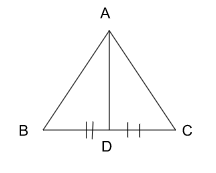c. The first quartile of a date is 70 and the third quartile is 25 more than double the first quartile, find the coefficient of the quartile deviation of the data.

## Group - c

1. Two function are f(x) =$\frac{2x+5}{8} and g(x) = 3x - 4$, if $(fog^{-1})(x)$ is an identity function, find the value of x.
2. The sum of three numbers in an AP is 21. If the second number is reduced by 1 and the third term is increased by 1, we obtain the GP. find the numbers.
3. Identify weather the function h(x) = $\frac{4x^{2}-25}{2x-5}$has continuity or discontinuity at x = 2.5
4. Solve by the matrix method = $\frac{3x+5y}{24}+\frac{5x-2y}{9}=\begin{bmatrix}3 & 2 \\4 & 3 \end{bmatrix}$
5. Find the equation of a pair of lines passing through the point (1, 0) and parallel to the lines represented by the equation  $3x^{2}+xy-10y^{2}=0$
6. Prove that :  $\sin^{2}(\frac{\pi^{c}}{4}-A)=\frac{(1-tanA)^{2}}{1+\tan^{2}A}$
7. If ∝ + β + θ = $\pi^{c}$ prove that. Sin (∝ + β - θ ) + sin (β+∝  - θ ) + sin (θ + ∝ - β) = 4 sin∝ sinβ sinθ
1. The angle of elevation of the top of the tower observed from two points on the same plane is found to be complementary. If the points are at a distance of 20m and 45m from the foot of the tower. Find the height of the tower.
1. Find 2🇽2 transformation matrix which transforms a square ABCD with vertices A(2, 3) C(4, 5), and D(2, 5) into a square A’B’C’D’ with vertices A’(2, 3), B’(4, 3) C’(5, 4) and D’(5, 2).
1. Find the interquartile range and coefficient of quartile deviation from the following data
 Weight (kg) 10 - 20 10 - 30 10 - 40 10 - 50 10 - 60 10 - 70 No.of students 12 31 31 36 46 61

1. Calculate the mean deviation from the median on the basis of the following data.
 Marks 10 - 20 20 - 30 30 - 40 40 - 50 50 - 60 60 - 70 Frequency 6 8 11 14 8 3

## GROUP D

1. Find the maximum and minimum values of the objective function p(x, y) = 16x - 2y under the following constraints: 3x + 5y  ≤ 24, 0  ≤ y ≤ 4 and 0 ≤ x ≤ 7
2. Find the equation of a circle passing through the points (4, 1) and (6, 5) and having its circle on line 4x + y = 16
3. Prove by vector method that the diagonal of a parallelogram bisects each other.
4. the coordinates of vertices of a quadrilateral ABCD are (1, 1), B(2, 3), C(4, 2), and D(3, 2). Rotate this quadrilateral about the origin through 180. reflect this image of quadrilaterals about y = - x, and write the name of the transformation which denotes the combined transformation of the above two transformations.

# Set - 3

## GROUP A

1

1. Define trigonometric function.
2. What does arithmetic mean between two numbers a and b?

2

1. Define the continuity of a function
2. If A =$\begin{bmatrix}a & b \\c & d \end{bmatrix}$, find |A|.

4

1. Express 2sinx cos y in the sum or difference of sine
2. If A + B + C = $\pi^{c}$, express cos(A + B) in terms of angle.

5

1. If the sum of position vectors of vertices of a triangle is $6\overrightarrow{i}+9\overrightarrow{j}$ then find the position vector of its centroid.
2. What will be the combined enlargement of two successive enlargements defined by $E_{1}[0_{1},k_{1}] and E_{2}[0_{2},k_{2}]?$

## GROUP B

6.

1. If $f^{-1}(x)= \frac{x-1}{3}$ then find f(x).
2. Find the sum of the first ‘n’ natural numbers.
3. Find the sum of series 9 + 3 + 1 …..+ $\frac{1}{27}$

7.

1. If an inverse of matrix A is $A^{-1}\begin{bmatrix}1 & 3 \\2 & 4 \end{bmatrix}$, find matrix A.
2. If A =$\begin{bmatrix}3 & 1 \\4 & 2\end{bmatrix}$  and B = $\begin{bmatrix}1 & 2 \\3& 5\end{bmatrix}$  find the value of |AB|.

8

1. If the lines 2x - 8y + 6 = 0 and px - 12y - 4 = 0 are parallel to each other. Find the value of p.
2. Find the equation of a circle having a center (1, - 2) and a diameter of $2\sqrt{5}$ units

9.

1. Prove that: $\frac{1+sinA-cosA}{1+sinA+\cos2A}=\tan\frac{A}{2}$
2. Prove that: $\cos20^{0}+\cos140^{o}-\cos100^{o}=0$
3. Solve $\sqrt{2}\tan\theta=3 [0^{o}\leq\theta\leq\pi^{c}]$

10.

1.  $\overline{a}+\overline{b}+\overline{c}=0, \overline{a}=6, \overline{b}= 10 and \overline{c} =14,$ find the angle between $\overline{a}and \overline{b}$.
2. The position vector of points A and B are $(8\overline{i}+6\overline{j})$ and $(3\overline{i}+\overline{j})$ respectively, find the position vector of point c, which divides AB in the ratio of 2:3 internally.
3. In the data, the value of the first quartile is ‘x’ and the quartile deviation is also (x). Find the third quartile and the coefficient of quartile deviation.

## Group - C

1. Solve:  $2x^{3} - 9x^{2} + 7x + 6 = 0$
2. Find the maximum value of z = 10x + 12y under the following constraints x + y ≤ 6, x - y ≤ 4, x≥ 0, and y ≥ 0.
3. Examine the continuity or discontinuity of the function f(x) = x+14x+4 at x = 4
4. Solve by cramer's rule 2x = 3(6 - y), 3x = 2y + 1
5. Find the separate equation of the pair of lines represented by the equation $6x^{2} + xy - y^{2}= 0.$ Also, find the angle between them.
6. Prove that: $2\sin^{2}A-\cos^{2}A.\cos2B=\sin^{2}B-cos^{2}B\cos2A$
7. If A + B + C = $\pi^{c}$, prove that:$2\sin^{2}A+cos^{2}B+\cos^{2}C-1$ = 4cosAcosBcosC + 1
8. The shadow of a column standing on a level plane is found to be 60m longer when the sun's altitude is $30^{o}$ than when it is $45^{o}$ proving that the height of the column is $30\sqrt{3}+1$m
9. The triangle$\begin{bmatrix}2 & 6&6 \\1 & 1&5\end{bmatrix}$ is transformed under the combined transformation$\begin{bmatrix}0 & 1 \\1 & 0\end{bmatrix}$ followed by,$\begin{bmatrix}0 & 1 \\-1 & 0\end{bmatrix}$ finding the final image.
10. Find the mean deviation from the mean of the data.
 x 0 - 10 10 - 20 20 - 30 30 - 40 40 - 50 f 2 3 4 5 6

1. Calculate the coefficient of variation from the data below
 Class interval 0 - 10 10 - 20 20 - 30 30 - 40 40 - 50 Frequency 2 3 4 5 6

## Group - D

1. the product of the first five terms of a geometric series is 243. If the third term of the geometric series is equal to the tenth term of an arithmetic series. Find the sum of the first 19 terms of the arithmetic series.
2. The equation of a circular design is  $2x^{2} + 2y^{2} - 28y + 98 = 0.$ Find the center, diameter, and area of the design.
3. In the figure, what type of triangle is ΔAOB then proved by the vector method that OC丄AB.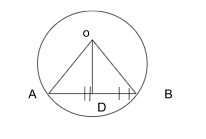1. A triangle ABC with vertices A(2, 3), B(2, 6) and C(3, 4) is translated by T = $\frac{-2}{3}$ and the image so obtained is enlarged by E[(0, 0), 2]. Write the coordinates of the vertices of the images so formed and represent ΔABC and both images in the same graph. Also, write the nature of images.

# Set- 4

## GROUP A

1.

1. What is the minimum value of y = cos x?
2. What is the nth arithmetic mean of an AS whose first term is ‘a’ and whose common difference is ‘d’

2.

1. What is meant by the interval (a, b)?
2. What should be the formula to calculate (x, y) Cramer’s rule when D, Dx, and Dy are given?

3.

1. A plane cuts the right cone not passing its vertex and semi-vertical angle a is less than the angle made by the plane to the axis of the cone. Name the section formed.
2. Write the formula to find the angle between the pair of the straight line represented by the equation $ax^{2}+2hxy+by^{2} = 0$

4.

1. What are multiple angles?
2. If A+B+C = $\pi^{c}$ express sin (B+A) in terms of angle C.

5.

1. State the section formula of external division.
2. If $T_{1}= \begin{bmatrix}a \\b \end{bmatrix}$ and $T_{2}= \begin{bmatrix} c \\d \end{bmatrix}$, what is the vector representing $T_{0}oT_{2}?$

## Group B

6.

1.  If F:X 3x+b and ff(2) = 12 find the value of b.
2. If a, a-2, and a+1 are in a geometric sequence. Find the value of a.
3. Draw (2x-1) $\frac{1}{2}$(x+15) on a graph and shade the region which represents its solutions.

7.

1. If E =$\begin{bmatrix}-3& 4 \\2 & -2a \end{bmatrix}$ and |E| = 22, find the value of a.
2. If the inverse of the matrix $\begin{bmatrix} 2x& 7 \\5 & 9 \end{bmatrix}$ is the matrix,$\begin{bmatrix} 9& y \\-5 & 4 \end{bmatrix}$  calculate the value of x and y.

8.

1. If the lines represented by the equation $9x^{2}+12xy+(k+1)y^{2} = 0$are coincident, find the value of k.
2. Find the equation of the circle having (2,3) and (4, 0) as the endpoints of a diameter.

9.

1. Prove that: $\sin^{4}B+\cos^{4}B=1-\frac{1}{2}\sin^{2}2B$
2. If $\cos\frac{B}{3}=\frac{1}{2}(m+\frac{1}{m})$ then prove that cosB $=\frac{1}{2}(m^{3}+\frac{1}{m^{3}})$
3. Solve for acute angle $2\sin^{2}\theta-3\sin\theta+1=0$

10.

1. For what value of x are the two vectors$33\overrightarrow{i}-2\overrightarrow{j}$ and $x\overrightarrow{i}-3\overrightarrow{j}$ perpendicular to each other?
2. In the figure if $\overline{P}A=\frac{1}{4}3\overline{p}+\overline{q}$
3. Prove that $\overline{a}= \frac{1}{4}(3\overline{p}+\overline{q}).$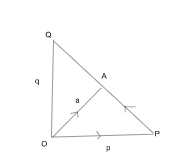The third quartile of the data is 15, if the coefficient of quartile deviation is 14, find the first quartiles and the inter-quartile range of the data.

## Group C

1. If f(x) = 2x+5, g(x) = $\frac{3x-1}{2}$ and $fog^{-1}(x)$= f(x) then find the value of x.
2. Solve graphically: $x^{2}-x-2= 0$
3. Prove that the function f(x) = $\begin{bmatrix}2x-1& x<2\\3 & x=2\\x+1&x>2 \end{bmatrix}$ is continuous at a point x = 2.
4. Solve by matrix method $\frac{3}{y}=\frac{2}{x}+2, \frac{6}{x}=\frac{2}{y} =2$
5. If an angle between the pair of lines represented by the equation  $2x^{2}+kxy+3y^{2}=0 is 45^{0}$, then find the positive value of k and also find the separate equations of the lines.
6. Prove that:$8sin20^{o}. Sin40^{o}. Sin 80^{o} = \sqrt{3}$
7. If A+B+C = $\pi^{c}$then prove that: sinA cosA+sinB cosB+ sinC cosC = 2sinA sinB sinC
8. The pole in one corner of a football ground of 10cm$\times$30cm is observed from the other corner which is opposite non-co-interior to the pole is $30^{o}$. Find the angle of the pole.
9. From the following data, find the mean deviation and its coefficient.
 Class interval 2-4 2-6 2-8 2-10 Frequency 4 9 11 12

1. Find the standard deviation from the following data.
 Class 12-18 22-28 32-38 42-48 52-58 Frequency 3 7 10 5 3

1. Find the inverse of the line segment joining the points A(8, 10)and B (8, 6) with respect to a circle with center C(4, 6) and a radius of 8 units. Show the inversion transformation in the graph.

## Group D

1. The expression $x^{2}+bx=c$ leaves the remainder when it is divided by (x+1) and when it is divided by (x-2), the remainder is 13. Find the values of b and c.
2. The vertices of a triangle are A(-2, 1), and B(2, 2). Find the equation of the altitude AD and median AP drawn from A to BC.
3. What is the centroid of a triangle? In $\Delta$PQR, QS is the median and G is the centroid. If O is the origin then prove that: $\overline{OG}= \frac{1}{3}(\overline{OP}+\overline{OQ}+\overline{OR})$
4. Take a right-angled $\Delta$ABC in a graph. Rotate the ABC through +$90^{o}$ about the center at the origin and then enlarge with the center at the origin and the scale factor 2. Find the image and show them on the same graph paper.

# Set -5

## GROUP A

1.

1. What is the y-intercept of y = tan x?
2. What is the sum of an n term of an arithmetic sequence whose first term is a and the lost term is b?

2.

1. Write the symbolic notation of the left-hand limit.
2. What type of equation can be solved using Cramer’s rule?

3.

1. A plane perpendicularly intersects a cone not passing through the vertex. Hence the section so formed.
2. The slope of a line $L_{1}is \frac{3}{4}$ find the slope of another line parallel to the line ($L_{1}$)

4.

1. Express 2 sec$\theta$. sin$\theta$ in the sum or difference of sin$\theta$
2. If cot x = tan x, what is the acute angle of x?

5.

1. If $\overline{a}-k\overline{b}$, what is the angle between $\overline{a} and \overline{b}$,  where k is a scalar quantity?
2. Where is the inverse of p if p is inside the circle of inversion?

## Group B

6.

1. If f(x) is a factor of f(x) = $x^{3}+kx^{2}-4x+12$ then find the value of k.
2. If 25, m+1, 35 is an arithmetic sequence then find the value of m.
3. What will be the point of intersection of the curve f(x) = $x^{2}-1$ and f(x)= 5?

7.

1.  If A =$\begin{bmatrix}2 & -1 \\3 & 1\end{bmatrix}$, find |A| and write whether $A^{-1}$ is defined or not.
2. If =$\begin{bmatrix}2p &7 \\-5 & 9 \end{bmatrix}$ -1, find the value of p.

8.

1. If two lines px+qy+r = 0 and lx-my+n = 0  are perpendicular to each other then show that Pl = qm.
2. Find the angle between the pair of the straight line represented by the equation $3x^{2}+7xy+2y^{2} = 0$

9.

1. Prove that: $cot \frac{A}{2}-tan \frac{A}{2} = 2 Cot A$
2. Prove that $\frac{\cos20^{o}-\sin20^{o}}{\cos20^{o}+\sin20^{o}}=\tan25^{o}$
3. Solve, $\sin2\theta+cos\theta= 0 [0^{o}]\leq\theta\geq90^{o}]$

10.

1.  If $\overline{a}= \begin{bmatrix}0 \\5 \end{bmatrix}$ and $\overline{b}= \begin{bmatrix}4\\4 \end{bmatrix}$find the angle between a and b
2.  If $\overrightarrow{O}A=\overrightarrow{a}, \overrightarrow{O}B=\overrightarrow{b}, \overrightarrow{O}M=\overrightarrow{m}$ and M divides BA internally in the ratio of 3:2 then prove that $\overrightarrow{m}=\frac{1}{5}(3\overrightarrow{a}+2\overrightarrow{b}).$
3. In continuous data, the value of the first quartile is two times the value of the quartile deviation. Find the coefficient of the quartile deviation.

## Group C

1. Solve: $x^{3}= 7x^{2}-36$
2. Find the sum of the first 9 terms of a geometric series whose third term and seventh term are 20 and 320 respectively.
3. Examine the continuity or discontinuity of f(x) =$\begin{cases}3-x & x \leq 0\\3 & x > 0\end{cases}at x = 0.$
4. Solve by matrix method $\frac{4}{x}+\frac{3}{y}=1, \frac{3}{x}-\frac{2}{y}= \frac{1}{24}$
5. Find the equation of the circle which passes through the points (3, 2) and (5, 4) and its center lies on the line 3x-2y-1 = 0
6. Prove that. $\cos^{3}\theta.\cos3\theta+\sin^{3}\theta.\sin3\theta = \cos^{3}2\theta$
7. If A +B+C = $\pi^{c}$, prove that: $\frac{cosA}{sinB.sinC}+\frac{cosB}{sinC.sinA}+\frac{cosC}{sinA.sinB}= cosec30^{o}$
8. At the foot of a mountain, the angle of elevation of its summit is$45^{o}$. After ascending 1km forwards at an angle of inclination $30^{o}$, the elevation changes to $60^{o}$. Find the height of the mountain.
9. E denotes enlargement about the center (-3, -4) with a scale factor of 2 and R denotes a reflection on the line y = 0, $\Delta$ABC having the vertices A (2, 0), B(3,1) and C(1, 1) is mapped under the combined transformation of EOR, find the image of $\Delta$ABC and draw two figure on the same graph paper.
10. Find QD (semi-interquartile range) and its coefficient.
 Marks 30-40 40-50 50-60 60-70 70-80 80-90 No. of students 10 5 3 5 2 2

1. Calculate the standard deviation of the data.
 Class interval 0-10 10-20 20-30 30-40 40-50 Frequency 2 6 5 4 3

## Group D

1. Solve graphically:$x^{2}-x-2 = 0$
2. Find two separate equations and the angle between them or represented by the second-degree equation$6x^{2}+xy-y^{2}-24x+8y = 0$
3. If $\overrightarrow{O}A = \overrightarrow{a}, \overrightarrow{O}B =\overrightarrow{b}$ and M divides the line segment AB externally in the ratio of m:n then prove that $\overrightarrow{O}M = \frac{m\overrightarrow{b}-n\overrightarrow{a}}{m-n}$.
4. P(-3, 2), Q(-1, 4) and R(2, 0) are the vertices of $\Delta$PQR. find the vertices of the image of the triangle under the reflection on the x-axis by using the matrix method.